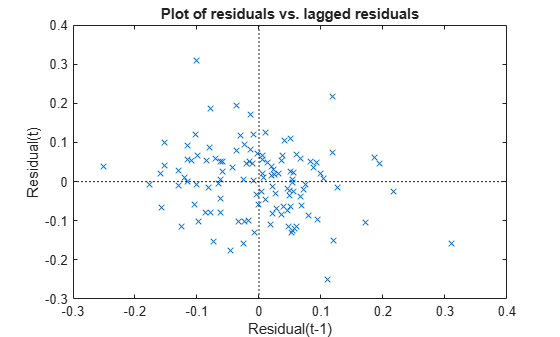# plotResiduals

Class: LinearMixedModel

Plot residuals of linear mixed-effects model

## Syntax

``plotResiduals(lme,plottype)``
``plotResiduals(lme,plottype,Name,Value)``
``h = plotResiduals(___)``

## Description

example

````plotResiduals(lme,plottype)` plots the raw conditional residuals of the linear mixed-effects model `lme` in a plot of the type specified by `plottype`.```

example

````plotResiduals(lme,plottype,Name,Value)` also plots the residuals of the linear mixed-effects model `lme` with additional options specified by one or more name-value pair arguments. For example, you can specify the residual type to plot.`plotResiduals` also accepts some other name-value pair arguments that specify the properties of the primary line in the plot. For those name-value pairs, see `plot`.```
````h = plotResiduals(___)` returns a handle, `h`, to the lines or patches in the plot of residuals.```

## Input Arguments

expand all

Linear mixed-effects model, specified as a `LinearMixedModel` object constructed using `fitlme` or `fitlmematrix`.

Type of residual plot, specified as one of the following.

 `'histogram'` Default. Histogram of residuals `'caseorder'` Residuals versus case (row) order `'fitted'` Residuals versus fitted values `'lagged'` Residuals versus lagged residual (r(t) versus r(t – 1)) `'probability'` Normal probability plot `'symmetry'` Symmetry plot

Example: `plotResiduals(lme,'lagged')`

### Name-Value Arguments

Specify optional pairs of arguments as `Name1=Value1,...,NameN=ValueN`, where `Name` is the argument name and `Value` is the corresponding value. Name-value arguments must appear after other arguments, but the order of the pairs does not matter.

Before R2021a, use commas to separate each name and value, and enclose `Name` in quotes.

Residual type, specified by the comma-separated pair consisting of `ResidualType` and one of the following.

Residual TypeConditionalMarginal
`'Raw'`

`${r}_{i}^{C}={\left[y-X\stackrel{^}{\beta }-Z\stackrel{^}{b}\right]}_{i}$`

`${r}_{i}^{M}={\left[y-X\stackrel{^}{\beta }\right]}_{i}$`

`'Pearson'`

`$p{r}_{i}^{C}=\frac{{r}_{i}^{C}}{{\sqrt{\left[{\stackrel{^}{Var}}_{y,b}\left(y-X\beta -Zb\right)\right]}}_{ii}}$`

`$p{r}_{i}^{M}=\frac{{r}_{i}^{M}}{\sqrt{{\left[{\stackrel{^}{Var}}_{y}\left(y-X\beta \right)\right]}_{ii}}}$`

`'Standardized'`

`$s{t}_{i}^{C}=\frac{{r}_{i}^{C}}{\sqrt{{\left[{\stackrel{^}{Var}}_{y}\left({r}^{C}\right)\right]}_{ii}}}$`

`$s{t}_{i}^{M}=\frac{{r}_{i}^{M}}{\sqrt{{\left[{\stackrel{^}{Var}}_{y}\left({r}^{M}\right)\right]}_{ii}}}$`

For more information on the conditional and marginal residuals and residual variances, see `Definitions` at the end of this page.

Example: `'ResidualType','Standardized'`

## Output Arguments

expand all

Handle to the residual plot, returned as a handle.

## Examples

expand all

`load('weight.mat')`

`weight` contains data from a longitudinal study, where 20 subjects are randomly assigned to 4 exercise programs, and their weight loss is recorded over six 2-week time periods. This is simulated data.

Store the data in a table. Define `Subject` and `Program` as categorical variables.

```tbl = table(InitialWeight,Program,Subject,Week,y); tbl.Subject = categorical(tbl.Subject); tbl.Program = categorical(tbl.Program);```

Fit a linear mixed-effects model where the initial weight, type of program, week, and the interaction between the week and type of program are the fixed effects. The intercept and week vary by subject.

`lme = fitlme(tbl,'y ~ InitialWeight + Program*Week + (Week|Subject)');`

Plot the histogram of the raw residuals.

`plotResiduals(lme)`Plot the residuals versus the fitted values.

`plotResiduals(lme,'fitted')`There is no obvious pattern, so there are no immediate signs of heteroscedasticity.

Create the normal probability plot of residuals.

`plotResiduals(lme,'probability')`Data appears to be normal.

Find the observation number for the data that appears to be an outlier to the right of the plot.

`find(residuals(lme)>0.25)`
```ans = 101 ```

Create a box plot of the raw, Pearson, and standardized residuals.

```r = residuals(lme); pr = residuals(lme,'ResidualType','Pearson'); st = residuals(lme,'ResidualType','Standardized'); X = [r pr st]; boxplot(X,'labels',{'Raw','Pearson','Standardized'})```All three box plots point out the outlier on the right tail of the distribution. The box plots of raw and Pearson residuals also point out a second possible outlier on the left tail. Find the corresponding observation number.

`find(pr<-2)`
```ans = 10 ```

Plot the raw residuals versus lagged residuals.

`plotResiduals(lme,'lagged')`There is no obvious pattern in the graph. The residuals do not appear to be correlated.